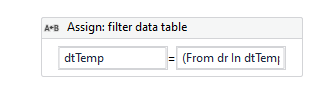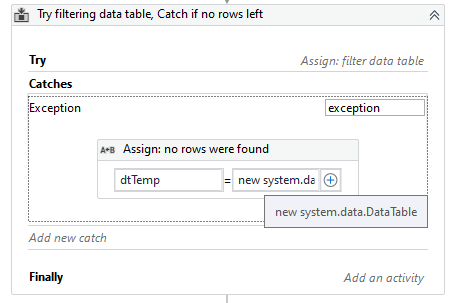# Need help/advice in resolving a select from datatable

Hi all,

My goal is to select all rows from a datatable based on a condition where i want to test if the value falls within a range that is calculated within the table itself.

I’m having difficulties writing out the correct .select for this. Right now i have this but i am getting an error still.

Can you check if u can see where it is wrong ?

mydatatablevariable.Select(“Convert([columnname1], ‘System.String’) ='”+stringvariable1 + “’ AND Convert([columnname2], ‘System.String’) ='”+ stringvariable2 + “'AND Convert([columname3containsInt], ‘System.Double’) >= '(Convert(”+ stringvariable3containingint + “, ‘System.Double’) - Convert([columnname4containingint], ‘System.Double’))'”).ToArray

My main issues is with comparing the value and converting them to doubles, how can i perform a calculation within the .select?

Hope u guys can help me out with this syntax, Thanks all.

Hi,

I think it’s simple and better to use LINQ as the following.

``````mydatatablevariable.AsEnumerable.Where(Function(r) r("columnname1").ToString = stringvariable1 AndAlso r("columnname2").ToString=stringvariable2 AndAlso Double.Parse(r("columnname3containingInt").ToString)>=Double.Parse(stringvariable3containingInt) AndAlso Double.Parse(r("columnname4containingInt").ToString)>=Double.Parse(stringvariable4containingInt)).ToArray
``````

Regards,

1 Like

Hello @Ewout

Instead of using .Select it might be more efficient to use LINQ methods.

You can do this as following:

Having the data table in memory (dtTemp) use an Assign activity:Where in the Value field enter the LINQ expression:

The expression means:
For all of the rows in dtTemp for each row check if it contains the conditions after `Where` word. Get the rows which meet the conditions and save them to the data table.
I used the intial data table (dtTemp), but you can of course save it to different variable.

Mind that if such LINQ expression returns empty data table, it throws an error, so it should be handled somehow, eg. put it in try catch:1 Like

Thanks @Yoichi and @Marta, i’ve combined both solutions and went with the following LINQ method:

datatablevariable.AsEnumerable.Where(Function(r) r(“Columnname1”).ToString = Variable1.tostring AndAlso (“Columnname2”).ToString=variable2.ToString AndAlso Double.Parse(r(“columnname3containingInt”).ToString)>= Double.Parse(variable3containingInt.ToString) AndAlso Double.Parse(variable3containingInt.tostring)>=(Double.Parse(r(“columnname3containingInt”).ToString) - Double.Parse(r(“columnname4containingInt”).ToString))).ToArray

I still have to test it within the rest of the process but in seperate tests it seems to be giving me the correct array of rows! very happy with this LINQ syntax! I made sure to include a try catch and in the catch I declare an empty array.

Effectively it is allowing me to select rows based on a range of values with an upper and lower limit. Checking if “variable3containingInt” falls within the range.

From now on I’m using LINQ2 Likes

This topic was automatically closed 3 days after the last reply. New replies are no longer allowed.